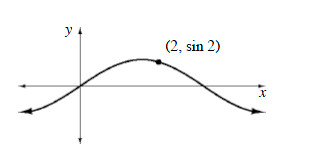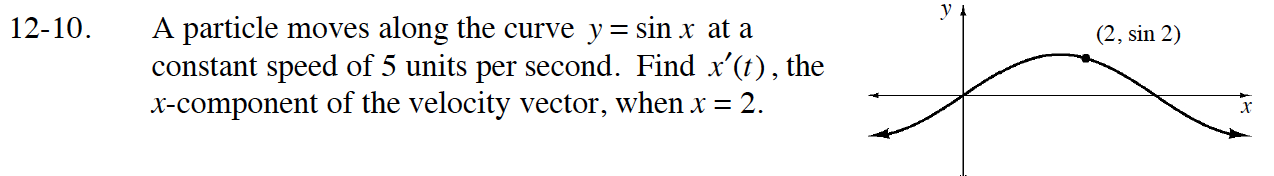### Home > CALC > Chapter 12 > Lesson 12.1.1 > Problem12-10

12-10.

A particle moves along the curve y = sin x at a constant speed of 5 units per second. Find x′(t), the x-component of the velocity vector, when x = 2. Homework Help ✎$5=\sqrt{(x^\prime(t))^2+(y^\prime(t))^2}\text{ or }25=(x^\prime(t))^2+(y^\prime(t))^2$

$25=(x^\prime(t))^2+(x^\prime(t)\cos(x))^2)$

$x^\prime(t)=\sqrt{\frac{25}{1-\cos^2(x)}}$

Evaluate x(2).